# Bihar Board Class 10th Maths Solutions Chapter 14 Statistics Ex 14.1

Bihar Board Class 10th Maths Solutions Chapter 14 Statistics Ex 14.1 Textbook Questions and Answers.

## BSEB Bihar Board Class 10th Maths Solutions Chapter 14 Statistics Ex 14.1Question 1.
A survey was conducted by a group of students as a part of their environment awareness programme, in which they collected the following data regarding the number of plants in 20 houses in a locality. Find the mean number of plants per house.Which method did you use for finding the mean, and why?
Solution:
Calculation of MeanWe have used direct method because numerical values of xi and fi are small.Question 2.
Consider the following distribution of daily wages of 50 workers of a factory :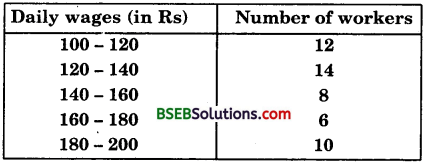Find the mean daily wages of the workers of the factory by using an appropriate method.
Solution:
Let the assumed mean, A = 150 and class-size, h = 20.
So, ui = $$\frac{x_{i}-\mathrm{A}}{h}$$ = $$\frac{x_{i}-150}{20}$$
We construct the table :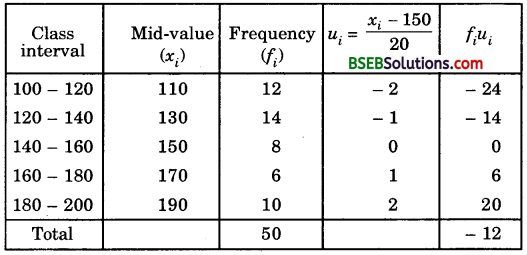$$\bar { E }$$ = A + h$$\frac{\Sigma f_{i} u_{i}}{\Sigma f_{i}}$$ = 150 + 20 x $$\frac { -12 }{ 50 }$$
= 150 – 4.8
= 145.2
Hence, mean = Rs 145.20

Question 3.
The following distribution shows the daily pocket allowdnce of children of a locality. The mean pocket allowance is Rs 18. Find the missing frequency f.Solution:
Let the assumed mean, A = 16, class size, h = 2,
So, ui = $$\frac{x_{i}-\mathrm{A}}{h}$$ = $$\frac{x_{i}-16}{2}$$We have : $$\bar { x }$$ = 18, A = 16 and h – 2.Hence, the missing frequency is 20.Question 4.
Thirty women were examined in a hospital by a doctor and the number of heart beats per minute were recorded and summarised as follows. Find the mean heart beats per minute for these women, choosing a suitable method.Solution:
Let the assumed mean, A = 75.5 and class size, h = 3.
So, ui = $$\frac{x_{i}-\mathrm{A}}{h}$$ = $$\frac{x_{i}-75.5}{3}$$
We construct the following table :So, $$\bar { x }$$ = A + h$$\frac{\Sigma f_{i} u_{i}}{\Sigma f_{i}}$$ = 75.6 + 3 x $$\frac { 4 }{ 30 }$$
= 75.5 + 0.4 = 75.9
Hence, the mean heart beats per minute for these women is 75.9.

Question 5.
In a retail market, fruit vendors were selling mangoes kept in packing boxes. These boxes contained varying number of mangoes. The following was the distribution of mangoes according to the number of boxes.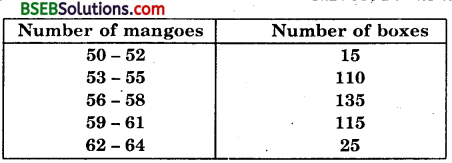Find the mean number of mangoes kept in a packing box. Which method of finding the mean did you choose?
Solution:
Here, the class intervals are formed by the exclusive method. If we make the data an inclusive one, the mid-values remain same. So, there is no need to convert the data with the caution that while finding h, we should count both the limits of class interval. For example, for 53 – 55, both 53 and 55 should be counted and thus h – 3 and not 55 – 53 = 2.
Let the assumed mean be A = 60 and h = 3.
So, ui = $$\frac{x_{i}-\mathrm{A}}{h}$$ = $$\frac{x_{i}-60}{3}$$
Calculation of Mean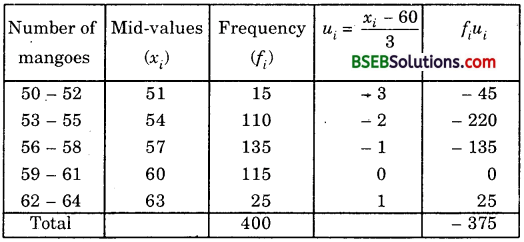∴ $$\bar { x }$$ = A + h$$\frac{\Sigma f_{i} u_{i}}{\Sigma f_{i}}$$ = 60 + 3 x $$\frac { -375 }{ 400 }$$
= 60 – 2.8125 = 57.1875 = 57.19 (nearly)
Hence, mean number of mangoes per box is 57.19.
Here, we have used the step-deviation method for finding the mean.

Question 6.
The table below shows the daily expenditure on food of 25 households in a locality.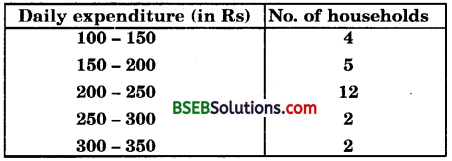Find the mean daily expenditure on food by a suitable method.
Solution:
Let the assumed mean, A = 225, class size, h = 50
So, ui = $$\frac{x_{i}-\mathrm{A}}{h}$$ = $$\frac{x_{i}-225}{50}$$
We construct the following table :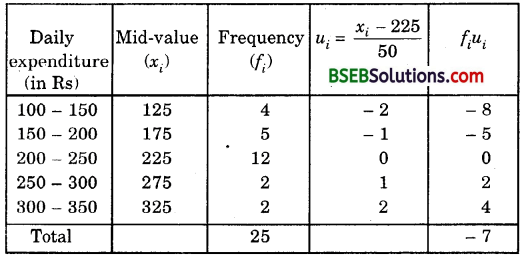So, $$\bar { x }$$ = A + h$$\frac{\Sigma f_{i} u_{i}}{\Sigma f_{i}}$$
= 225 + 50 x $$\frac { -7 }{ 25 }$$
= 225 – 14 = 211
Hence, the mean daily expenditure on food is Rs 211.Question 7.
To find the concentration of SO2 in the air (in parts per million, i.e., ppm), the data was collected for 30 localities in a certain city and is presented below :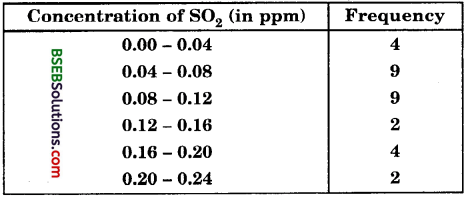Find the mean concentration of SO2 in the air.
Solution:
Calculation of mean by direct methodQuestion 8.
A class teacher has the following absentee record of 40 students of a class for the whole term. Find the mean number of days a student was absent.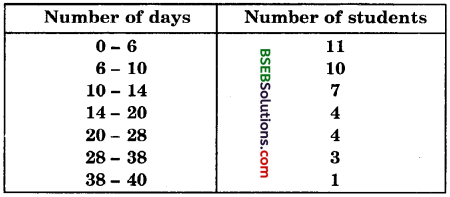Solution:
Here, the class size varies, and xi’s are small.
Let us apply the direct method here :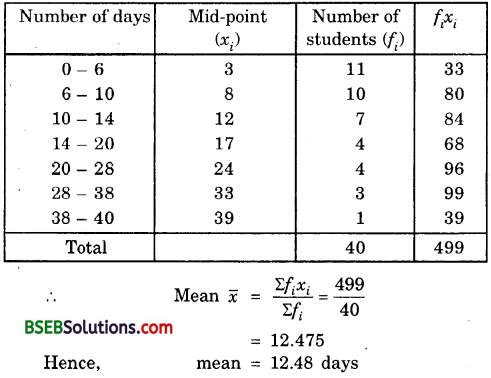Question 9.
The following table gives the literacy rate (in percentage) of 35 cities. Find the mean literacy rate.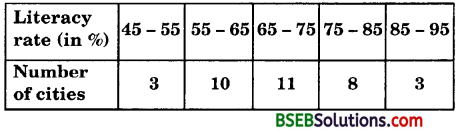Solution:
Let the assumed mean, A = 70 and class size, h = 10.
So, ui = $$\frac{x_{i}-\mathrm{A}}{h}$$ = $$\frac{x_{i}-70}{10}$$Thus, mean literacy rate is 69.43%.